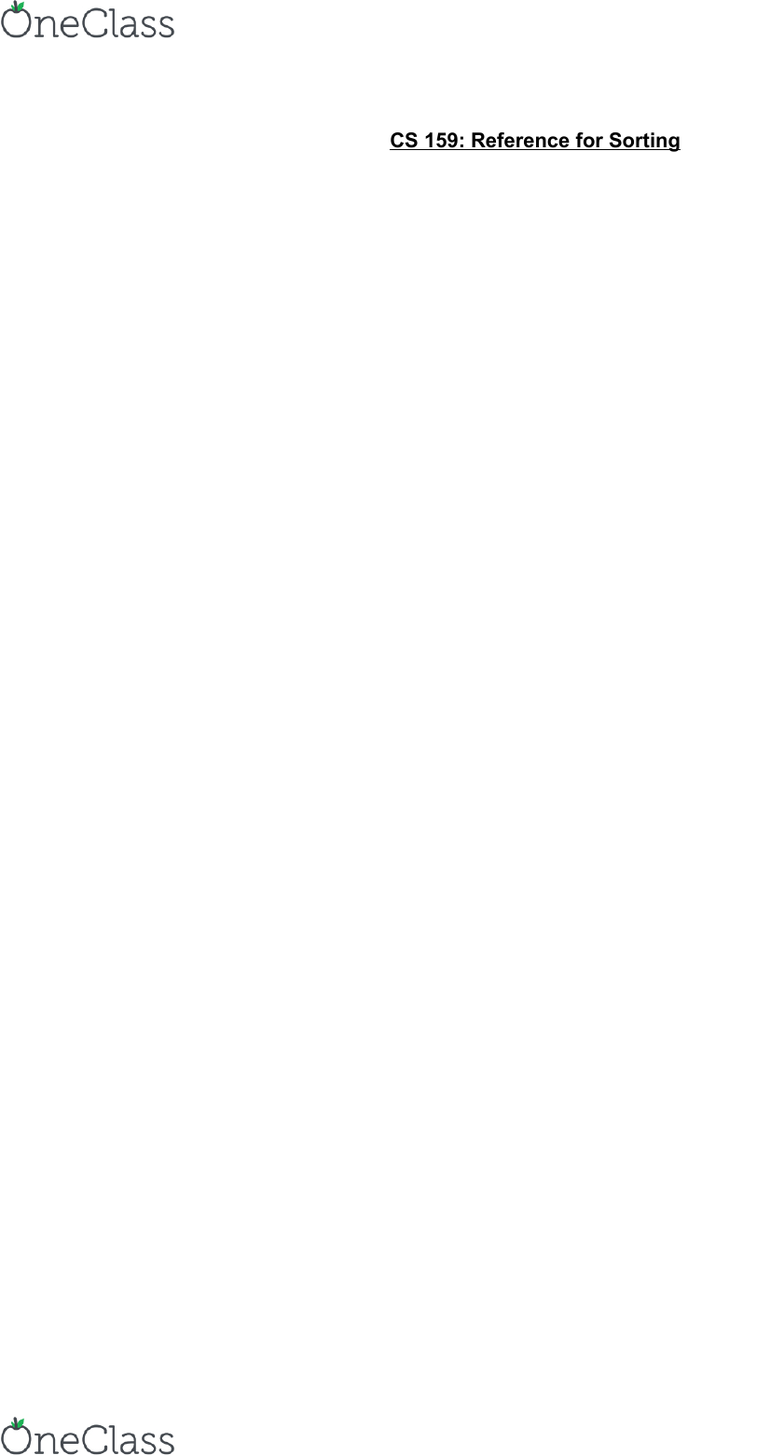Textbook Notes (280,000)
US (110,000)
Purdue (700)
CS (30)
Chapter 8

# CS 15900 Chapter Notes - Chapter 8: Bubble Sort, Insertion Sort, Selection Sort

Department
Computer Sciences
Course Code
CS 15900
Professor
Alan Bunning
Chapter
8

Page:
of 1CS 159: Reference for Sorting
Bubble Sort: repeatedly steps through the list, compares each pair of adjacent elements, and
swaps them if they’re in the wrong order
**stable, O(1) extra space, O(n^2) comparisons and swaps, Adaptive: O(n) when nearly sorted
*Algorithm:
for i = 1:n,
swapped = false
for j = n:i+1,
if a[j] < a[j-1],
swap a[j,j-1]
swapped = true
→ invariant: a[1..i] in final position
break if not swapped
end
Insertion Sort: steps through the list, takes next unsorted element and places it in the
appropriate place within the sorted data
**stable, O(1) extra space, O(n^2) comparisons and swaps, adaptive: O(n) time when nearly
*Algorithm:
for i = 2:n,
for (k = i; k > 1 and a[k] < a[k-1]; k--)
swap a[k,k-1]
→ invariant: a[1..i] is sorted
end
Selection Sort: steps through the list, finds the smallest value in the unsorted list and moves it
to the sorted list after the previous smallest value
**not stable, O(1) extra space, Θ(n^2) comparisons, Θ(n) swaps, not adaptive
*Algorithm:
for i = 1:n,
k = i
for j = i+1:n, if a[j] < a[k], k = j
→ invariant: a[k] smallest of a[i..n]
swap a[i,k]
→ invariant: a[1..i] in final position
end
find more resources at oneclass.com
find more resources at oneclass.com